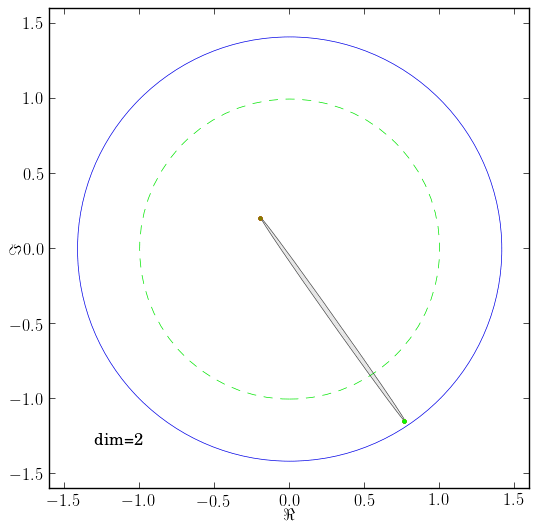# Numerical range and spectrum of random Ginibre matrix

Let $G$ be a matrix of $\mathrm{dim}\ G=1000$ drawn from Ginibre ensemble and let $G_d$ be a family of matrices such that $G_d=P_d(T)$, where $T$ is upper triangular matrix obtained by Schur decomposition of $G$ such that $G=UTU^\dagger$. $P_d$ are orthogonal projections $P_d(\cdot)=\sum_{i=1}^d \ketbra{i}{l_i} \cdot \ketbra{l_i}{i}$, where $l_i$ is a sequence of integers from $1$ to $1000$. $G_d$ are normalized so $\tr G_d G_d^\dagger=\mathrm{dim} G_d$.

In the figure red dots indicate spectrum of $G_d$, gray area is numerical range $W(G_d)$, green circle has radius $1$ outer circle has radius $\sqrt{2}$.